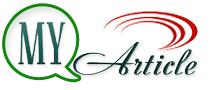# Economic Order Quantity (EOQ)

Understanding Economic Order Quantity is the number of products to be ordered to meet the supply. Of course, a number of products purchased must meet an economic value. EOQ should be able to minimize variable costs. Included in the variable cost in this case is the cost of storage and ordering costs.

We can imagine that if the number exceeds the number of product units booking reservation economies, this will make the cost of storage is higher than the inventory cost of the reservation economy. Furthermore, when the number of units of the product ordering of the booking amount which is less economical, the booking fee will be greater than the cost of ordering of the booking amount which is economical. This is because the company had to order a product many times with booking fee multiplied.

Costs include the cost of renting warehouse storage, electricity costs, taxes, insurance, and others. While fees may include costs between goods from the warehouse ordering, inspection costs, material handling costs, and others. In the EOQ models, these costs are calculated on an annual basis.

Other components included in the model is the EOQ reorder point (reorder point). Reorder point is a point (a certain item) in which company to order again. Reorder Point depending on the lead time, the time it takes the company to meet the order. So, EOQ models should also be able to answer the question how much and when the items to be ordered in order to achieve an economical value.

In general, the model calculations (formula) EOQ is as follows.

Description:

Q * = value of EOQ (unit)
C = booking fee per order
R = requests per year (unit)
h = holding cost